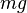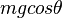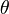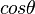# Normal force facts for kids

Kids Encyclopedia Facts

Normal force is the force that the ground (or any surface) pushes back up with. If there was no normal force, you'd be slowly seeping into the ground.

The normal force on a object is always perpendicular (at a right angle) to the surface the object is on.

On a flat surface, the normal force of an object is$mg$ (the object's weight, that is its mass multiplied by the force of gravity).

On an inclined plane, the normal force is reduced by the angle, and the normal force is$mgcos\theta$.

Note that on a flat surface,$\theta$ would be 0, and so$cos\theta$ would be 1. Thus, the two equations are equal.

## Images for kidsNormal force Facts for Kids. Kiddle Encyclopedia.